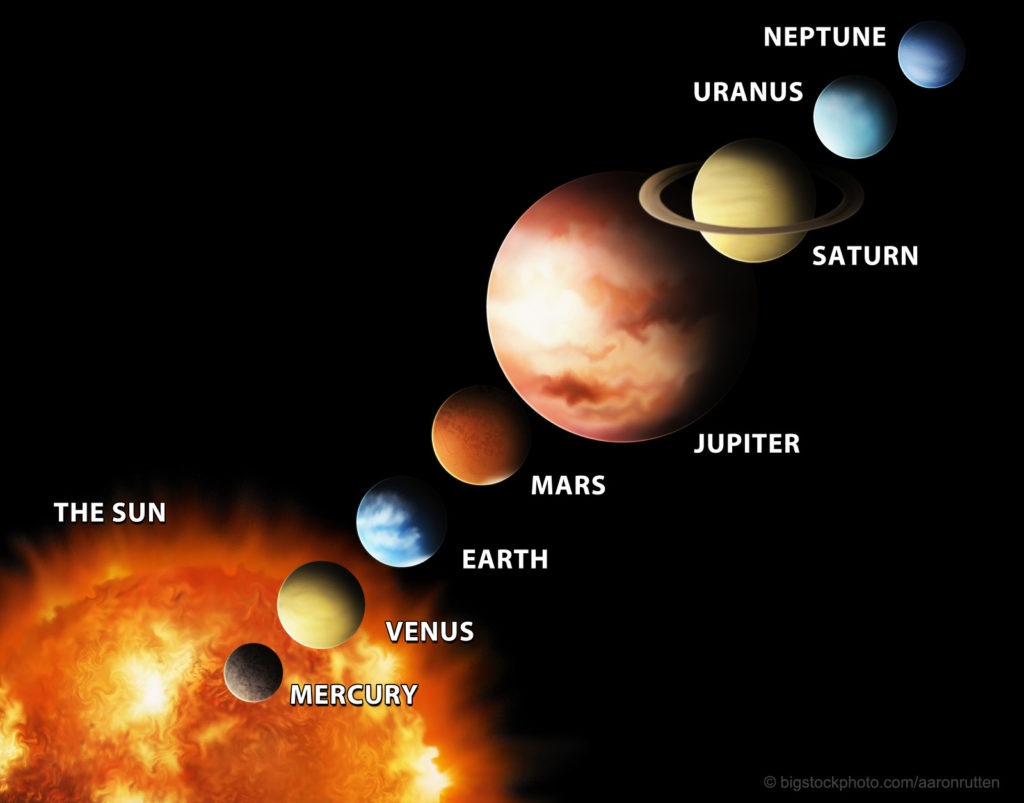# Chaos Mathematics and Solar System DesignOne of the ongoing mysteries about the solar system is the question of how the orbits of the planets and other objects function. If the solar system consisted of only the Sun and the Earth, the motion of the Earth could be easily calculated by a simple formula. When you add other planets and moons, all tugging and pulling on Earth in different ways at different times, no simple mathematical formula explains what is taking place. The solar system seems to be incredibly stable, but why should that be? Chaos mathematics helps us see design in the solar system.

For hundreds of years, astronomers and mathematicians have wrestled with the questions that relate to the consistency of the solar system. Why does Mars not get pulled out of its orbit and crash into Earth? Why does Earth not drift closer to the Sun when it is pulled on by the gravitation of the inner planets? How does the Moon’s gravity affect Earth’s orbit and rotation?

Modern computers have given us spectacular advances in understanding planetary motions. The simple calculation gets complicated when you add a second planet to the solar system. With three objects tugging on each other and the Earth no longer follows a precisely elliptical orbit. Earth experiences different gravitational pulls at different times depending on the distances between the objects. With each planet, moon, and even asteroid the calculations become more difficult.

Since no simple formula accurately describes the planetary motions, French astronomer Jacques Lasker and others have used an advanced technique called chaos mathematics. The term chaos in this application does not mean a disorderly system. Chaos refers to situations in which the behavior of a dynamic system depends sensitively on the initial variables that control the final outcome. In this case, each of the planetary gravitational effects is written as an equation called a differential equation. By carrying all of the equations out to include many variables and then averaging the equations, Lasker was able to describe the orbits very successfully. Other scientists have found that adding other influences such as the effects of relativity increase the accuracy and predicted stability of Earth’s orbit.

The importance of this work is that it shows why the solar system consists of many objects and not just the Earth and Sun. A resonant system of gravitational forces is needed to keep the stability and consistency of our orbit around the Sun. Chaos theory and the use of computers that can do incredibly complicated calculations have opened the door to a better understanding of our complex solar system.

Romans 1:19-22 tells us that we can know that there is a God through the things He has made. Psalms 19:1 and Isaiah 40:26 tell us to examine the heavens and see the handiwork of God. Chaos mathematics tells us that the initial state is crucial to the outcome. God established the initial state which has given us our present stable solar system.

The more we learn of the creation, the more we learn of the Creator. Chaos theory in mathematics shows us the wisdom and planning built into the orbit of our planet around the Sun.